# 高端函数## Curried functions

``````ghci> max 4 5
5
ghci> (max 4) 5
5
````````````multThree :: (Num a) => a -> a -> a -> a
multThree x y z = x * y * z
``````

``````ghci> let multTwoWithNine = multThree 9
ghci> multTwoWithNine 2 3
54
ghci> let multWithEighteen = multTwoWithNine 2
ghci> multWithEighteen 10
180
``````

``````compareWithHundred :: (Num a, Ord a) => a -> Ordering
compareWithHundred x = compare 100 x
``````

``````compareWithHundred :: (Num a, Ord a) => a -> Ordering
compareWithHundred = compare 100
``````

Yo! 你得保证已经弄明白了 Curried functions 与不全调用的原理，它们很重要！

``````divideByTen :: (Floating a) => a -> a
divideByTen = (/10)
``````

``````isUpperAlphanum :: Char -> Bool
isUpperAlphanum = (`elem` ['A'..'Z'])
``````

``````ghci> multThree 3 4
:1:0:
No instance for (Show (t -> t))
arising from a use of `print' at :1:0-12
Possible fix: add an instance declaration for (Show (t -> t))
In the expression: print it
In a 'do' expression: print it
``````

ghci 说，这一表达式回传了一个 `a -> a` 型别的函数，但它不知道该如何显示它。 函数不是 `Show` 型别类的实例，所以我们不能得到表示一函数内容的字串。 若在 ghci 中计算 `1+1`，它会首先计算得 `2`，然后调用 `show 2` 得到该数值的字串表示，即 `"2"`，再输出到屏幕.

## 是时候了，来点高端函数！

``````applyTwice :: (a -> a) -> a -> a
applyTwice f x = f (f x)
````````````*Note*: 现在开始我们会直说某函数含有多个参数(除非它真的只有一个参数)。 以简洁之名，我们会说 ``(a->a->a)`` 取两个参数，尽管我们知道它在背后做的手脚.
``````

``````ghci> applyTwice (+3) 10
16
ghci> applyTwice (++ " HAHA") "HEY"
"HEY HAHA HAHA"
ghci> applyTwice ("HAHA " ++) "HEY"
"HAHA HAHA HEY"
ghci> applyTwice (multThree 2 2) 9
144
ghci> applyTwice (3:) 
[3,3,1]
``````

``````zipWith' :: (a -> b -> c) -> [a] -> [b] -> [c]
zipWith' _ [] _ = []
zipWith' _ _ [] = []
zipWith' f (x:xs) (y:ys) = f x y : zipWith' f xs ys
``````

``````ghci> zipWith' (+) [4,2,5,6] [2,6,2,3]
[6,8,7,9]
ghci> zipWith' max [6,3,2,1] [7,3,1,5]
[7,3,2,5]
ghci> zipWith' (++) ["foo "，"bar "，"baz "] ["fighters"，"hoppers"，"aldrin"]
["foo fighters","bar hoppers","baz aldrin"]
ghci> zipWith' (*) (replicate 5 2) [1..]
[2,4,6,8,10]
ghci> zipWith' (zipWith' (*)) [[1,2,3],[3,5,6],[2,3,4]] [[3,2,2],[3,4,5],[5,4,3]]
[[3,4,6],[9,20,30],[10,12,12]]
``````

``````flip' :: (a -> b -> c) -> (b -> a -> c)
flip' f = g
where g x y = f y x
``````

``````flip' :: (a -> b -> c) -> b -> a -> c
flip' f y x = f x y
``````

``````ghci> flip' zip [1,2,3,4,5] "hello"
[('h',1),('e',2),('l',3),('l',4),('o',5)]
ghci> zipWith (flip' div) [2,2..] [10,8,6,4,2]
[5,4,3,2,1]
``````

## map 与 filter

map 取一个函数和 List 做参数，遍历该 List 的每个元素来调用该函数产生一个新的 List。 看下它的型别声明和实现:

``````map :: (a -> b) -> [a] -> [b]
map _ [] = []
map f (x:xs) = f x : map f xs
``````

``````ghci> map (+3) [1,5,3,1,6]
[4,8,6,4,9]
ghci> map (++ "!") ["BIFF"，"BANG"，"POW"]
["BIFF!","BANG!","POW!"]
ghci> map (replicate 3) [3..6]
[[3,3,3],[4,4,4],[5,5,5],[6,6,6]]
ghci> map (map (^2)) [[1,2],[3,4,5,6],[7,8]]
[[1,4],[9,16,25,36],[49,64]]
ghci> map fst [(1,2),(3,5),(6,3),(2,6),(2,5)]
[1,3,6,2,2]
``````

filter 函数取一个限制条件和一个 List，回传该 List 中所有符合该条件的元素。它的型别声明及实现大致如下:

``````filter :: (a -> Bool) -> [a] -> [a]
filter _ [] = []
filter p (x:xs)
| p x       = x : filter p xs
| otherwise = filter p xs
``````

``````ghci> filter (>3) [1,5,3,2,1,6,4,3,2,1]
[5,6,4]
ghci> filter (==3) [1,2,3,4,5]

ghci> filter even [1..10]
[2,4,6,8,10]
ghci> let notNull x = not (null x) in filter notNull [[1,2,3],[],[3,4,5],[2,2],[],[],[]]
[[1,2,3],[3,4,5],[2,2]]
ghci> filter (`elem` ['a'..'z']) "u LaUgH aT mE BeCaUsE I aM diFfeRent"
ghci> filter (`elem` ['A'..'Z']) "i lauGh At You BecAuse u r aLL the Same"
"GAYBALLS"
``````

``````quicksort :: (Ord a) => [a] -> [a]
quicksort [] = []
quicksort (x:xs) =
let smallerSorted = quicksort (filter (<=x) xs)
biggerSorted = quicksort (filter (>x) xs)
in  smallerSorted ++ [x] ++ biggerSorted
``````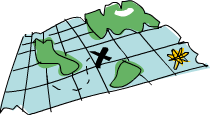`map``filter` 是每个函数式进程员的面包黄油(呃，`map``filter` 还是 List Comprehension 并不重要)。 想想前面我们如何解决给定周长寻找合适直角三角形的问题的? 在命令式编程中，我们可以套上三个循环逐个测试当前的组合是否满足条件，若满足，就打印到屏幕或其他类似的输出。 而在函数式编程中，这行就都交给 `map``filter`。 你弄个取一参数的函数，把它交给 `map` 过一遍 List，再 `filter` 之找到合适的结果。 感谢 Haskell 的惰性，即便是你多次 `map` 一个 ``List` 也只会遍历一遍该 List，要找出小于 100000 的数中最大的 3829 的倍数，只需过滤结果所在的 List 就行了.

``````largestDivisible :: (Integral a) => a
largestDivisible = head (filter p [100000,99999..])
where p x = x `mod` 3829 == 0
``````

``````ghci> sum (takeWhile (<10000) (filter odd (map (^2) [1..])))
166650
``````

``````ghci> sum (takeWhile (<10000) [m | m <- [n^2 | n <- [1..]], odd m])
166650
``````

``````chain :: (Integral a) => a -> [a]
chain 1 = 
chain n
| even n =  n:chain (n `div` 2)
| odd n  =  n:chain (n*3 + 1)
``````

``````ghci> chain 10
[10,5,16,8,4,2,1]
ghci> chain 1

ghci> chain 30
[30,15,46,23,70,35,106,53,160,80,40,20,10,5,16,8,4,2,1]
``````

yay! 貌似工作良好。 现在由这个函数来告诉我们结果:

``````numLongChains :: Int
numLongChains = length (filter isLong (map chain [1..100]))
where isLong xs = length xs > 15
``````

``````*Note*: 这函数的型别为 ``numLongChains :: Int``。这是由于历史原因，``length`` 回传一个 ``Int`` 而非 ``Num`` 的成员型别，若要得到一个更通用的 ``Num a``，我们可以使用 ``fromIntegral`` 函数来处理所得结果.
``````

`map`，我们可以写出类似 `map (*) [0..]` 之类的代码。 如果只是为了例证 Curried functions 和不全调用的函数是真正的值及其原理，那就是你可以把函数传递或把函数装在 List 中(只是你还不能将它们转换为字串)。 迄今为止，我们还只是 `map` 单参数的函数到 List，如 `map (*2) [0..]` 可得一组型别为 `(Num a) => [a]` 的 List，而 `map (*) [0..]` 也是完全没问题的。`*` 的型别为 `(Num a) => a -> a -> a`，用单个参数调用二元函数会回传一个一元函数。如果用 `*``map` 一个 `[0..]` 的 List，就会得到一组一元函数组成的 List，即 `(Num a) => [a->a]``map (*) [0..]` 所得的结果写起来大约就是 `[(0*),(1*),(2*)..]`.

``````ghci> let listOfFuns = map (*) [0..]
ghci> (listOfFuns !! 4) 5
20
``````

## lambda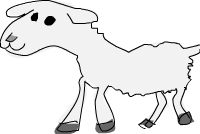lambda 就是匿名函数。有些时候我们需要传给高端函数一个函数，而这函数我们只会用这一次，这就弄个特定功能的 lambda。编写 lambda，就写个 `\` (因为它看起来像是希腊字母的 lambda -- 如果你斜视的厉害)，后面是用空格分隔的参数，`->` 后面就是函数体。通常我们都是用括号将其括起，要不然它就会占据整个右边部分。

``````numLongChains :: Int
numLongChains = length (filter (\xs -> length xs > 15) (map chain [1..100]))
``````lambda 是个表达式，因此我们可以任意传递。表达式 `(\xs -> length xs > 15)` 回传一个函数，它可以告诉我们一个 List 的长度是否大于 15。

``````ghci> zipWith (\a b -> (a * 30 + 3) / b) [5,4,3,2,1] [1,2,3,4,5]
[153.0,61.5,31.0,15.75,6.6]
``````

``````ghci> map (\(a,b) -> a + b) [(1,2),(3,5),(6,3),(2,6),(2,5)]
[3,8,9,8,7]
``````

``````addThree :: (Num a) => a -> a -> a -> a
addThree x y z = x + y + z
``````
``````addThree :: (Num a) => a -> a -> a -> a
addThree = \x -> \y -> \z -> x + y + z
``````

``````flip' :: (a -> b -> c) -> b -> a -> c
flip' f = \x y -> f y x
``````

## 关键字 fold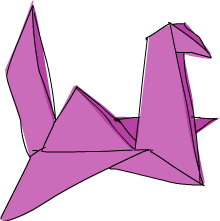``````sum' :: (Num a) => [a] -> a
sum' xs = foldl (\acc x -> acc + x) 0 xs
``````

``````ghci> sum' [3,5,2,1]
11
``````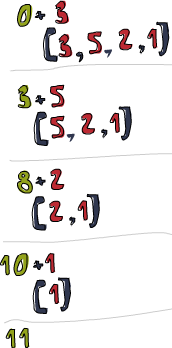``````sum' :: (Num a) => [a] -> a
sum' = foldl (+) 0
``````

``````elem' :: (Eq a) => a -> [a] -> Bool
elem' y ys = foldl (\acc x -> if x == y then True else acc) False ys
``````

``````map' :: (a -> b) -> [a] -> [b]
map' f xs = foldr (\x acc -> f x : acc) [] xs
``````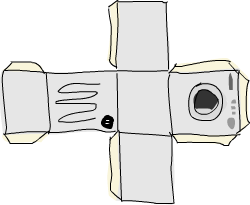foldl1foldr1 的行为与 `foldl``foldr` 相似，只是你无需明确提供初始值。他们假定 List 的首个(或末尾)元素作为起始值，并从旁边的元素开始折叠。这一来，`sum` 函数大可这样实现：`sum = foldl1 (+)`。这里待折叠的 List 中至少要有一个元素，若使用空 List 就会产生一个运行时错误。不过 `foldl``foldr` 与空 List 相处的就很好。所以在使用 `fold` 前，应该先想下它会不会遇到空 List，如果不会遇到，大可放心使用 `foldr1``foldl1`

``````maximum' :: (Ord a) => [a] -> a
maximum' = foldr1 (\x acc -> if x > acc then x else acc)

reverse' :: [a] -> [a]
reverse' = foldl (\acc x -> x : acc) []

product' :: (Num a) => [a] -> a
product' = foldr1 (*)

filter' :: (a -> Bool) -> [a] -> [a]
filter' p = foldr (\x acc -> if p x then x : acc else acc) []

head' = foldr1 (\x _ -> x)

last' :: [a] -> a
last' = foldl1 (\_ x -> x)
``````

scanlscanr`foldl``foldr` 相似，只是它们会记录下累加值的所有状态到一个 List。也有 scanl1scanr1

``````ghci> scanl (+) 0 [3,5,2,1]
[0,3,8,10,11]
ghci> scanr (+) 0 [3,5,2,1]
[11,8,3,1,0]
ghci> scanl1 (\acc x -> if x > acc then x else acc) [3,4,5,3,7,9,2,1]
[3,4,5,5,7,9,9,9]
ghci> scanl (flip (:)) [] [3,2,1]
[[],,[2,3],[1,2,3]]
``````

``````sqrtSums :: Int
sqrtSums = length (takeWhile (<1000) (scanl1 (+) (map sqrt [1..]))) + 1
``````
``````ghci> sqrtSums
131
ghci> sum (map sqrt [1..131])
1005.0942035344083
ghci> sum (map sqrt [1..130])
993.6486803921487
``````

`scan` 可以用来跟踪 `fold` 函数的执行过程。想想这个问题，取所有自然数的平方根的和，寻找在何处超过 1000？ 先`map sqrt [1..]`，然后用个 `fold` 来求它们的和。但在这里我们想知道求和的过程，所以使用 `scan``scan` 完毕时就可以得到小于 1000 的所有和。所得结果 List 的第一个元素为 1，第二个就是 1+根2，第三个就是 1+根2+根3。若有 `x` 个和小于 1000，那结果就是 `x+1`

## 有\$的函数调用

``````(\$) :: (a -> b) -> a -> b
f \$ x = f x
```````sum (filter (> 10) (map (*2) [2..10]))` 该如何？嗯，`\$` 是右结合，`f (g (z x))``f \$ g \$ z x` 等价。所以我么可以将 `sum (filter (> 10) (map (*2) [2..10])` 重写为 `sum \$ filter (> 10) \$ map (*2) [2..10]`

``````ghci> map (\$ 3) [(4+),(10*),(^2),sqrt]
[7.0,30.0,9.0,1.7320508075688772]
``````

## Function composition

``````(.) :: (b -> c) -> (a -> b) -> a -> c
f . g = \x -> f (g x)
````````````ghci> map (\x -> negate (abs x)) [5,-3,-6,7,-3,2,-19,24]
[-5,-3,-6,-7,-3,-2,-19,-24]
``````

``````ghci> map (negate . abs) [5,-3,-6,7,-3,2,-19,24]
[-5,-3,-6,-7,-3,-2,-19,-24]
``````

``````ghci> map (\xs -> negate (sum (tail xs))) [[1..5],[3..6],[1..7]]
[-14,-15,-27]
``````

``````ghci> map (negate . sum . tail) [[1..5],[3..6],[1..7]]
[-14,-15,-27]
``````

``````sum' :: (Num a) => [a] -> a
sum' xs = foldl (+) 0 xs
``````

``````fn x = ceiling (negate (tan (cos (max 50 x))))
``````

``````fn = ceiling . negate . tan . cos . max 50
``````

`map``filter` 那节中，我们求了小于 10000 的所有奇数的平方的和。如下就是将其置于一个函数中的样子：

``````oddSquareSum :: Integer
oddSquareSum = sum (takeWhile (<10000) (filter odd (map (^2) [1..])))
``````

``````oddSquareSum :: Integer
oddSquareSum = sum . takeWhile (<10000) . filter odd . map (^2) \$ [1..]
``````

``````oddSquareSum :: Integer
oddSquareSum =
let oddSquares = filter odd \$ map (^2) [1..]
belowLimit = takeWhile (<10000) oddSquares
in  sum belowLimit
``````